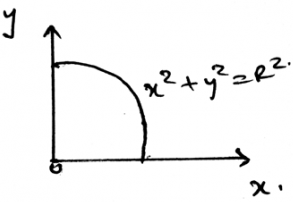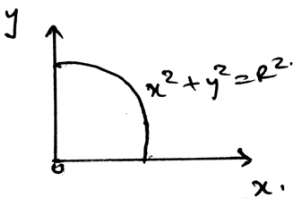Courses

# Test: Centroid Of A Body

## 15 Questions MCQ Test Engineering Mechanics | Test: Centroid Of A Body

Description
This mock test of Test: Centroid Of A Body for Mechanical Engineering helps you for every Mechanical Engineering entrance exam. This contains 15 Multiple Choice Questions for Mechanical Engineering Test: Centroid Of A Body (mcq) to study with solutions a complete question bank. The solved questions answers in this Test: Centroid Of A Body quiz give you a good mix of easy questions and tough questions. Mechanical Engineering students definitely take this Test: Centroid Of A Body exercise for a better result in the exam. You can find other Test: Centroid Of A Body extra questions, long questions & short questions for Mechanical Engineering on EduRev as well by searching above.
QUESTION: 1

### Density is best given by _______

Solution:

The density is the ratio of the mass to the volume of the body. Thus arranging them and the volume is said to be as ratio of mass to density. This equation is generally used in the calculations of the centroid of the body.

QUESTION: 2

### If solving the question in 3D calculations is difficult, then use the 2D system and then equate the ratio of the product of the centroid of the section to its mass to the total mass of the body to the centroid.

Solution:

The answer is obviously no. Still if we are having any difficulty in making the vector components, then we can go in 2D. As if the particle is in equilibrium, the net force will be zero. No matter where you see first. Net force is zero. But the statement here is not correct about the centroid.

QUESTION: 3

### One of the use of the centroid is as in the simplification of the loading system the net force acts at the ___________ of the loading body.

Solution:

In the simplification of the loading system the net force acts at the centroid of the loading body. That is if the loading system is in the form of the triangle then the at the distance 2 by 3 of the base the net force of the loading will act. And the load will be half the area of the loading.

QUESTION: 4

The use of centroid comes in picture as if the non-Uniform loading is of the type of parabola then what will be the best suited answer among the following?

Solution:

The net force will act at the centroid of the parabola. Whether it be a parabola or the cubic curve the centroid is the only point at which the net force act. Force can’t be acted horizontally if the loading is vertical. Hence whatever be the shape of the loading, the centroid is the point of action of net force. Thus the use of centroid.

QUESTION: 5

If any external force also is applied on the distributed loading then?

Solution:

The external forces are treated differently. They are not added by the force of the distributed loading. That is the force not only acts at the centroid always. It can be shifted also. Depending on the external forces. Thus the use of centroid.

QUESTION: 6

The x axis coordinate and the y axis coordinate of the centroid are having different types of calculations to calculate them.

Solution:

The calculation is general to all the axis of coordinate system. This means that the calculations are generalised for all the axis. All the axis are given same priority. Thus no change in calculations.

QUESTION: 7

The centre of ____________ is the ratio of the product of centroid and volume to the total volume.

Solution:

The product of the centroid of the section to its volume to the total volume of the body is the centre of volume. Thus the answer. The ratio is generally used to locate the coordinate of the centroid or the centre of volume.

QUESTION: 8

If the force vector F acting along the centroid is having its x-axis component being equal to Z N, y-axis component be X N and z-axis component be Y N then vector F is best represented by?

Solution:

It is given that x, y and z-axis components are Z, X and Y respectively. Thus, just by placing the right coordinates we get option d to be correct. Here just the interchange of the axis’s representor with their respective axis is creating confusion. Which must be figured out.

QUESTION: 9

Centroid of a body does depends upon the small weights of tiny particles. Which statement is right for force acting by the small particles of the body having it’s vector form as = Ai + Bj + Ck?

Solution:

As given the vector is F = Ai + Bj + Ck, this implies that the x ,y and z-axis components of this vector is A, B and C respectively. But, in rectangular components representation of any vector, the vector is written as F = Fx + Fy + Fz.

QUESTION: 10

Determine the y coordinate of centroid of the area in the shape of circle as shown.Solution:

The weight of the body is the sum of the all the small weights that are been applied by all the particles. The smaller the particle the smaller the weight it applies. Thus the total weight of the body is the summation of all the infinite number of small weights applied to the body.

QUESTION: 11

Centroid determination involves the calculations of various forces. In that forces are having various properties. That is force is developed by a support that not allows the ________ of its attached member.

Solution:

The force developed by a support doesn’t allow the translation of its attached member. This is the basic condition for the equilibrium of the forces in any dimension. This rule is applied when the support reactions are taken into the account for the equilibrium of the body.

QUESTION: 12

What is not the condition for the equilibrium for the calculations used for the determination of the centroid in three dimensional system of axis?

Solution:

For the equilibrium in the three dimensional system of axis we have all the conditions true as, ∑Fx=0, ∑Fy=0 and ∑Fz=0. Also we have the summation of the forces equal to zero. Which is not a non-zero value.

QUESTION: 13

The supports in the 3D are having more than three reaction forces. Because they are having three axis on which the components of the forces need to be zero.

Solution:

As 3D is defined as the three axis system, we have to consider the equilibrium in all the three axis. This will make the equilibrium go on all the axis of the 3D space. And hence will cancel all the forces. These are the points to be kept in considerence while determining the centroid.

QUESTION: 14

If any body is tied to three or more ropes, and then is allowed to achieve its equilibrium. Then the equilibrium achieved is achieved w.r.t what?

Solution:

Yes, the equilibrium is being achieved w.r.t the ground. Like the motion w.r.t ground need be zero. That is the relative velocity of the object or the body must be zero w.r.t the ground. This means motion is in equilibrium. This equilibrium is also defined by the centroid axis.

QUESTION: 15

Determine the x coordinate of centroid of the area in the shape of circle as shown.Solution:

The weight of the body is the sum of the all the small weights that are been applied by all the particles. The smaller the particle the smaller the weight it applies. Thus the total weight of the body is the summation of all the infinite number of small weights applied to the body.